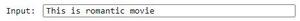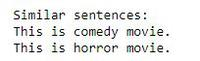# Find most similar sentence in the file to the input sentence | NLP

• Last Updated : 26 Nov, 2020

In this article, we will find the most similar sentence in the file to the input sentence.

Example:

Attention geek! Strengthen your foundations with the Python Programming Foundation Course and learn the basics.

To begin with, your interview preparations Enhance your Data Structures concepts with the Python DS Course. And to begin with your Machine Learning Journey, join the Machine Learning - Basic Level Course

```File content:
"This is movie."
"This is romantic movie"
"This is a girl."

Input: "This is a boy"

Similar sentence to input:
"This is a girl", "This is movie".```

Approach:

1. Create a list to store all the unique words of the file.
2. Convert all the sentences of the file into the binary format by comparing each word with the content of the list, after cleaning(removing stopword, stemming, etc.)
3. Convert the input sentence in the binary format.
4. Find the number of similar words in the input sentence to each sentence and store the value in the list named similarity index.
5. Find the maximum value of similarity index and return the sentence having maximum similar words.

Content of the file:Code to get a similar sentence:

## Python3

 `from` `nltk.stem ``import` `PorterStemmer``from` `nltk.tokenize ``import` `word_tokenize, sent_tokenize``import` `nltk``from` `nltk.corpus ``import` `stopwords`` ` ` ` `nltk.download(``'stopwords'``)``ps ``=` `PorterStemmer()``f ``=` `open``(``'romyyy.txt'``)``a ``=` `sent_tokenize(f.read())`` ` `# removal of stopwords``stop_words ``=` `list``(stopwords.words(``'english'``))`` ` `# removal of punctuation signs``punc ``=` `'''!()-[]{};:'"\, <>./?@#\$%^&*_~'''``s ``=` `[(word_tokenize(a[i])) ``for` `i ``in` `range``(``len``(a))]``outer_1 ``=` `[]`` ` `for` `i ``in` `range``(``len``(s)):``    ``inner_1 ``=` `[]``     ` `    ``for` `j ``in` `range``(``len``(s[i])):``         ` `        ``if` `s[i][j] ``not` `in` `(punc ``or` `stop_words):``            ``s[i][j] ``=` `ps.stem(s[i][j])``             ` `            ``if` `s[i][j] ``not` `in` `stop_words:``                ``inner_1.append(s[i][j].lower())``     ` `    ``outer_1.append(``set``(inner_1))``rvector ``=` `outer_1[``0``]`` ` `for` `i ``in` `range``(``1``, ``len``(s)):``    ``rvector ``=` `rvector.union(outer_1[i])``outer ``=` `[]`` ` `for` `i ``in` `range``(``len``(outer_1)):``    ``inner ``=` `[]``     ` `    ``for` `w ``in` `rvector:``         ` `        ``if` `w ``in` `outer_1[i]:``            ``inner.append(``1``)``         ` `        ``else``:``            ``inner.append(``0``)``    ``outer.append(inner)``comparison ``=` `input``(``"Input: "``)`` ` ` ` `check ``=` `(word_tokenize(comparison))``check ``=` `[ps.stem(check[i]).lower() ``for` `i ``in` `range``(``len``(check))]`` ` ` ` `check1 ``=` `[]``for` `w ``in` `rvector:``    ``if` `w ``in` `check:``        ``check1.append(``1``)  ``# create a vector``    ``else``:``        ``check1.append(``0``)`` ` `ds ``=` `[]`` ` `for` `j ``in` `range``(``len``(outer)):``    ``similarity_index ``=` `0``    ``c ``=` `0``     ` `    ``if` `check1 ``=``=` `outer[j]:``        ``ds.append(``0``)``    ``else``:``        ``for` `i ``in` `range``(``len``(rvector)):`` ` `            ``c ``+``=` `check1[i]``*``outer[j][i]`` ` `        ``similarity_index ``+``=` `c``        ``ds.append(similarity_index)`` ` ` ` `ds``maximum ``=` `max``(ds)``print``()``print``()``print``(``"Similar sentences: "``)``for` `i ``in` `range``(``len``(ds)):`` ` `    ``if` `ds[i] ``=``=` `maximum:``        ``print``(a[i])`

Output:My Personal Notes arrow_drop_up